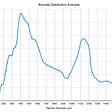# Data Science with the Penguins Data Set: Conditional Probability in Python

This blog is part of my series on doing / learning data science using the penguins data set.

## Goal

Over the years, I have encountered manuscripts, documentation, and blogs which mention that something is calculated, but it is not clear how it is calculated. In this post, I want to share with you:

• How to estimate conditional probability using pandas and numpy
• Some of the properties of conditional probability

## Intro

Conditional probability is easy to understand intuitively. For example:

• What are the chances of having a diseases knowing that my diagnostic test is positive ?
• Will I get to work if I don’t take the usual bus route ?
• What are the chances of a student being admitted to an educational institution if her/his/their SAT score is within a given range ?

On the other hand, the mechanics of computing a conditional probability are not that obvious (at least they were not obvious to me).

## Notation

Formula 1 at the top of this post already contains a description in English for each component; Thus, P(A | B) = P(A and B) / P(B) is the conditional probability distribution for A and B. For example, if A and B are each random variables with two possible values:

• A = {a1, a2}
• B = {b1, b2}

We could write four different conditional probablities based on Formula 1:

• P(A= a1| B= b1) = P(A= a1 and B= b1) / P(B= b1)
• P(A= a1| B= b2) = P(A= a1 and B= b2) / P(B= b2)
• P(A= a2| B= b2) = P(A= a2 and B= b2) / P(B= b2)
• P(A= a2| B= b1) = P(A= a2 and B= b1) / P(B= b1)

In the next section, I will share with you how to estimate conditional probabilities using Python.

## Python Code

The conditional probability distribution of A and B is calculated as follows:

• estimate the joint probability distribution P(A,B)
• estimate the conditonal probability distribution P(A |B) from P(A,B)

Get and clean some data:

Joint probability distribution

With the function above, we can calculate the joint probability distribution (JPD) for species and island:

For the table above A = species, and B = island. Thus, P(A=Adelie and B=Biscoe) = 0.128 = 12.8 %.

## Conditional probability distribution

The conditional probability distribution can be estimated as follows:

which leads to the following results for island and species (chosen in the JPD function):

The conditional probability distribution above (CPD) indicates the following:

P(A(Species) = a1 (Gentoo) | B(Island) = b1 (Biscoe) )= 0.738 = 73.8 %

using a more compact notation we could also say that:

P( S = Adelie | I = Torgersen ) = 100 %

P( S = Gentoo | I = Torgersen ) = 0 %

P( S = Chinstrap | I = Dream ) = 54.8%

A very interesting (and not obvious) property of conditional probabilities is that they are not symmetrical, which means that P(A|B) is not necessarily the same than P(B|A). We can see this property in our data by using the same function after transposing the JPD table:

P(A(Island) = a1 (Biscoe) | B(Species) = b1 (Gentoo) )= 1.00 = 100 %

while :

P( I = Torgersen | S = Adelie ) = 34.2 %

P( I = Torgersen | S = Gentoo ) = 0 %

P( I = Dream | S = Chinstrap) = 100%

## Conditional Expectations

Tables 2 and 3 also allow us to estimate the conditional expectation for each value of B = {b1,b2,b3}; as you can guess, this conditional expectation is not symmetrical.

When A = Species and B = Island, the conditional expectation for B = Biscoe (b1) is calculated as follows:

E(A | b1) = a1 P(A =a1 | b1) + a2 P(A =a2 | b1) + a3 P(A =a3 | b1)

where the levels of species are assigned arbitrary values as follows:

• a2 (Chinstrap) = 2
• a3 (Gentoo) = 3

Therefore:

E(A | b1) = 1 * 0.262+ 2 * 0.00 + 3 * .739 = 2.48

The remaining two conditional expectations are the following:

E(A | b2) = 1 *0.452 + 2*0.548 + 3*0 = 1.55

E(A | b3) = 1 * 1 + 2*0+ 3*0 = 1.0

## Source Code

The source code for this post is available below:

## More from Julio Cárdenas-Rodríguez

Scientist, modeling geek, immigrant, father, writer-at-heart, scientifically religious, and many other contradictions.

Love podcasts or audiobooks? Learn on the go with our new app.

## Fitting a Base (Trend Capturing) — MMM Modelling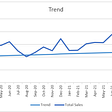## Case Study — House Price Prediction using Advanced Regresssion## The journey of “DATA”## Making cool maps using census data in 5 easy steps## How Averages Go Awry## Data science-Statistic Analysis(part 2)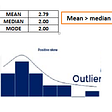## This Week in Data Preparation (November 16, 2020)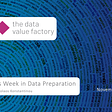## VTA Liberates Its Data from the Stone Age with Swiftly## Julio Cárdenas-Rodríguez

Scientist, modeling geek, immigrant, father, writer-at-heart, scientifically religious, and many other contradictions.

## Techniques to handle missing values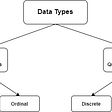## PCA: The Principal Component of Machine Learning## Multiple Linear Regression Explained## Kernel Density Estimation in Python [Part 1/2]